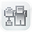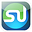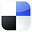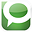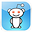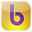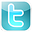Excel
STATISTICAL
Master

Other Useful Statistical Distribution

Clear and Complete - WITH LOTS OF SOLVED PROBLEMS

# Other Useful Distributions

Lots of Worked-Out, Easy-To-Understand, Graduate-Level Problems  --->
( Scroll Down and Take a Look ! )

Introduction

In additional to the Normal, t, and Binomial distributions, there are a number of other useful distributions that are commonly used to solve statistical problems that occur in business.

# Multinomial Distribution

The Multinomial Distribution is a generalization of the well-known Binomial Distribution.

Like the Binomial Distribution, the Multinomial Distribution is a Discrete distribution, not a Continuous distribution. This means that the objects that form the distribution are whole, individual objects. This distribution curve is not smooth but moves abruptly from one level to the next in increments of whole units.

The Multinomial Distribution provides the probability of a combination of specified outputs for a given number of trials that are totally independent. The probability of each of the individual outputs of each of the trials must be known in order to utilize the Multinomial Distribution to calculate the probability of that unique combination of outputs occurring in the given trials.

Here is the formula for calculating the probability of a multinomial distribution:

P ( X1 = n1,  X2 = n2,  …  ,  Xk = nk ) =

=
[ (n!) / (n1! * n2! * … *nk!) ] * [Pr(X1 = n1)]n1 * [Pr(X2 = n2)]n2 * ...

... *
[Pr(Xk = nk)]nk

The following example makes the Multinomial Distribution easier to understand.

Problem: A box contains 5 red marbles, 4 white marbles, and 3 blue marbles. A marble is selected at random, its color noted, and then the marble is replaced. 6 marbles are selected in this manner. Find the probability that out of those 6 marbles,  3 are red, 2 are white, and 1 is blue.

Total number of marbles = 12

n = total number of drawings = 6

X1 = Count of red marbles drawn = n1 = 3

X2 = Count of white marbles drawn = n2 = 2

X3 = Count of blue marbles drawn = n3 = 1

The probability of 3 red, 2 white, 1 blue = P(3 red, 2 white, 1 blue)

= P ( X1 = n1, X2 = n2, …, Xk = nk )

= P ( X1 = 3, X2 = 2, X3 = 3 )

P ( X1 = n1,  X2 = n2,  …  ,  Xk = nk ) =

=
[ (n!) / (n1! * n2! * … *nk!) ] * [Pr(X1 = n1)]n1 * [Pr(X2 = n2)]n2 * ...

... *
[Pr(Xk = nk)]nk

= [ (6!) / (3! * 2! * 1! ) ] * [5/12]3 * [4/12]2 * [3/12]1

=  625 / 5184  =  0.12056  =  12.06%

There is a 12.06% chance that, from a box containing 5 red marbles, 4 white marbles, and 3 blue marbles, from 6 marbles randomly selected,  3 are red, 2 are white, and 1 is blue.

This same problem above is solved in the Excel Statistical Master with only 1 Excel formula. Everything is explained to you in SIMPLE language in the Excel Statistical Master.

When k = 2, the Multinomial Distribution is the Binomial Distribution.

# Hypergeometric Distribution

The Hypergeometric Distribution is almost the same as the Binomial Distribution, except that samples are NOT replaced back into the population. This is known as Sampling Without Replacement.

The Binomial Distribution calculates the probability of 1 of 2 possible outcomes occurring a certain number of times (x) in a certain number of independent trials (n). The probability of the outcome occurring in a single trial is known (p).

After each trial the samples ARE replaced back into the population when using the Binomial Distribution.

The Hypergeometric Distribution calculates the probability of 1 of 2 possible outcomes occurring a certain number of times (x) in a certain number of independent trials (n). The probability of the outcome occurring   in a single trial is known (p).

After each trial the samples are NOT replaced back into the population when using the Hypergeometric Distribution.

The problem below will illustrate the use of the Hypergeometric Distribution formula:

Problem: A 20-piece chocolate sample consists of 8 caramel samples and 12 nut samples. Calculate the probability that of 4 individual samples 3 taken will produce caramels. Each sample is eaten (not replaced) after it is taken. (If each sample were replaced before the next sample was taken, the Binomial distribution would be used)

Exact number of successes = 3

Number of trials = 4

Initial possible number of successes = 8

Initial population size = 20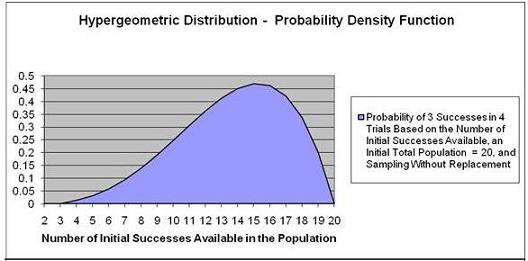There is a 13.87% probability that 3 out of 4 samples taken without replacement will be caramel samples if the box initially had 20 pieces of candy that included 8 caramel samples.

The y-value corresponding to the point on the horizontal axis equaling 8 is 13.87%.

This same problem above is solved in the Excel Statistical Master with only 1 Excel formula. The Excel Statistical Master is the fastest way for you to climb the business statistics learning curve.

Sampling WITH Replacement –

Using the Binomial Distribution

Problem: If the above problem were the same except that you were sampling with replacement (putting each candy back instead of eating it before the next sample is taken), it would solved with the Binomial Distribution as follows:

This problem is solved using the Probability Density Function and not the Cumulative Distribution Function because we are solving for exactly 3 caramels chosen in 4 trials, not up to 3 caramels.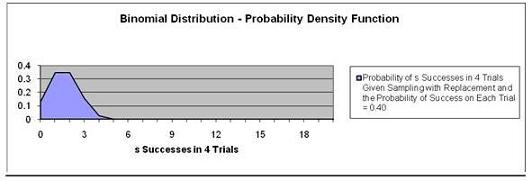There is 15.36% chance of exactly 3 successes in 4 trials given Sampling with Replacement and the Probability of Success on each trial is 0.40.

Note that the graph at point 3 on the Horizontal has a y value (probability) = 0.1536.

This same problem above is solved in the Excel Statistical Master with only 1 Excel formula. The Excel Statistical Master teaches you everything in step-by-step frameworks. You'll never have to memorize any complicated statisical theory.

# Poisson Distribution

The Poisson Distribution is a widely employed distribution that is used to describe the probability of events that are a result of a rate that occurs over time such as:

Product demand
Demand for services
Number of telephone calls that come over a switchboard
Number of accidents
Number of traffic arrivals
Number of defects

The Poisson Distribution is a Discrete distribution. This means that the events described by this function occur in whole units. The graph of the Poisson Distribution therefore moves from one level to the next in discrete increments, not smoothly.

The Poisson Distribution is used to calculate the probability of a certain number of specific events occurring over a given period of time - if it is known in advance that those events occur in frequency as predicted by the Poisson Distribution. Previous measurement must have been taken to determine: 1) that the events occur in frequency according to the Poisson Distribution, and 2) the average rate, which is the expected number of occurrences of that event over the given time period.

A problem will better illustrate the use of the Poisson Distribution:

Problem: An average of 4.8 telephone calls per minute is made through the central switchboard according to the Poisson distribution. What is the probability that:

a) exactly 4 phone calls will be made in a given minute

Exact number of events = k = 4

Expected number of events = E(k)

Cumulative Distribution Function? No, Use the Probability Density Function.

We want to calculate the probability of EXACTLY 4 phone calls. This will be the
Probability Density Function, not the Cumulative Distribution Function, which
would measure the probability of up to 4 phone calls instead of exactly 4.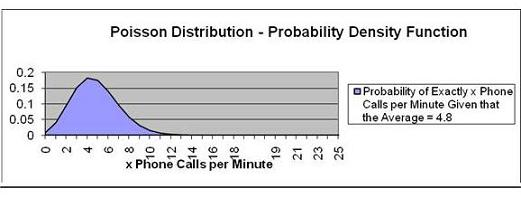There is an 18.2% chance of exactly 4 phone calls per minute.

Note that the point on the graph that has 4 as the value on the horizontal axis has
a value of 0.182 on the vertical axis. The Poisson distribution is a discrete distribution and not a continuous distribution so the graph has corners at each point instead of being smooth.

b) up to 4 phone calls will be made in a given minute

Exact number of events = k = 4

Expected number of events = E(k)

Cumulative Distribution Function? Yes

We want to calculate the probability of UP TO 4 phone calls. This will be the
Cumulative Distribution Function.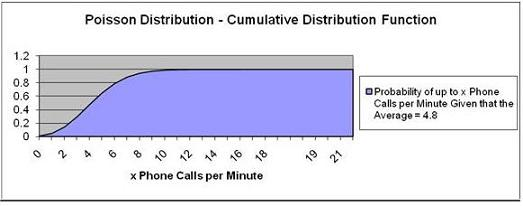Pr (Up To 4 Phone Calls) = Pr ( k = 4) = 47.6%

There is a 47.6% chance of up to 4 phone calls per minute.

Note that the point that represents up to 4 calls per minute corresponds with 0.47 on the vertical axis.

This same Poisson problems as above are each solved in the Excel Statistical Master with only 1 Excel formula. With the Excel Statistical Master you'll never have to look up anything on a chart ever again.

# Uniform Distribution

A variable is uniformly distributed if all possible outcomes of that variable have an equal probability of occurring. For example, if a fair die has 6 possible outcomes when rolled once, each outcome has the same 1/6 chance of occurring.

The Uniform Distribution is a Discrete distribution. This means that its events described by this function occur in whole units.

Problem: A fair die is rolled once. What is the probability that either a 2 or a 5 will appear on top after the roll?

Number of total possible outcomes in 1 trial = 6

Number of times that 2 appears as a possible outcome = 1

Number of times that 5 appears as a possible outcome = 1

Probability of a 2 occurring in 1 roll = 1/6 = 0.1667

Probability of a 2 occurring in 1 roll = 1/6 = 0.1667

Pr (2 occurs) OR Pr (5 occurs) = Pr (2 occurs) + Pr (5 occurs)

= 0.1667 + 0.1667 = 0.333 = 33.33% probability

There is a 33.33% probability that either a 2 or a 5 will appear on top after the roll.

# Exponential Distribution

The Exponential Distribution is used to calculate the probability of occurrence of an event that is the result of a continuous decaying or declining process. The lengths between arrival times in a Poisson process could be described with the
Exponential Distribution. Examples of arrival times between Poisson events are as follows:

Time between telephone calls that come over a switchboard
Time between accidents
Time between traffic arrivals
Time between defects

An example of a decaying process that would be predicted by the Exponential Distribution would be:

Time until a radioactive particle decays

The Exponential Distribution is not appropriate for predicting failure rates of devices or lifetimes of organisms because a disproportionately high number of failures occur in the very young and the very old. In these cases, the distribution
curve would not be a smooth exponential curve as described by the Exponential Distribution.

The Exponential Distribution predicts time between Poisson events as follows:

Probability of length of time t between Poisson events = f(t) = e-kt
k is sometimes called Lambda.

A problem will illustrate the use of this function:

Problem: A production machine has a very low defect rate. Time between defects can be predicted by the following Exponential Distribution function:

Time between failures (t) = f(t) = 9 e-9t
( t is measured in whole years )

Calculate the probability of a defect being produced within the next 1/10th year.

(Using "Within" indicates that the Cumulative Distribution function will be used)

t = 1/10 = 0.10

k = Lambda = 9

Use Cumulative Distribution Function?  Yes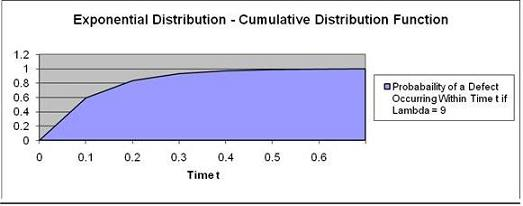The probability of defect occurring in 1/10 years  is 59.34%

Note that the graph point at Time t = 0.1 has the probability of 0.5934.

# Gamma Distribution

The Gamma Distribution represents the sum of n exponentially distributed random variables. Applications of the Gamma Distribution are often based on intervals
between Poisson-distributed events. Examples of these would include queuing models, the flow of items through manufacturing and distribution processes, and the load on web servers and many forms of telecom.

Due to its moderately skewed profile, it can be used as a model in a range of disciplines, including climatology where it is a working model for rainfall, and financial services where it has been used for modeling insurance claims and the size of loan defaults. It has therefore been used in probability of ruin and value-at-risk equations.

The Gamma Distribution function is characterized by 2 variables, its shape parameter, alpha (α), and its scale parameter, theta (Φ). The Gamma Distribution function calculates the probability of wait time between Poisson distributed events to be time t,

Problem: Calculate the probability of the a Poisson-distributed event occurring before Time t = 10 if the Gamma Distribution function has alpha, α, = 2 and theta, Φ, = 4.

Units of waiting time until event occurs = t = 10

Alpha, α = 2

Theta, Φ = 4

Use the Cumulative Distribution Function? Yes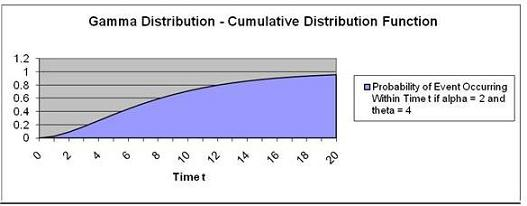The probability of this event occurring within 10 units of time = 71.27%

Note that the graph point at Time t = 10 has a probability of 0.7127.

This same problem above is solved in the Excel Statistical Master with only 1 Excel formula. The Excel Statistical Master will make you a fully functional statistician at your workplace.

The Chi-Squared Distribution is a Gamma distribution in which the shape parameter, α, is set to the degrees of freedom divided by 2 and the scale parameter, Φ, is set to 2.

The Gamma Distribution with its shape parameter, α, set to 1 and its scale parameter, Φ, set to b, becomes the Exponential Distribution with k, lambda, set to b.

# Beta Distribution

The Beta Distribution models events which are constrained to take place between a minimum and maximum time limit. For this reason, the Beta Distribution is often used for modeling project planning and control systems such as PERT (Project Evaluation and Review Technique) and CPM (Critical Path Method). The Beta Distribution is often used to calculate the probability that a project will be completed within a given period of time. Below is an example which illustrates its use:

Problem: Calculate the probability of completing the following project before Time t = 5 if the project is described by the following parameters:

Evaluation time period = t = 5

Alpha, α, = 8

Beta, ß, = 10

Minimum completion time in units of time = 2

Maximum completion time in units of time = 7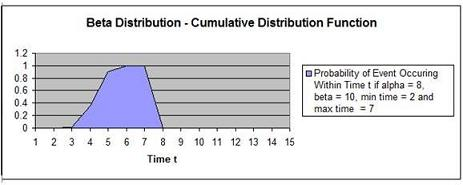The probability of completing the task within time = 5 units (within is a cumulative function) = 90.81%

Note that the graph point at Time t = 5 has a probability of 0.908.

This same problem above is solved in the Excel Statistical Master with only 1 Excel formula. With the Excel Statistical Master you can do advanced business statistics without having to buy and learn expensive, complicated statistical software packages such as SyStat, MiniTab, SPSS, or SAS.

# Weibull Distribution

The Weibull Distribution is a special case of the Generalized Extreme Value distribution. The Weibull distribution has been used extensively as a model of time to failure for manufactured items and has become one of the principal tools of reliability engineering. The applications of the Weibull Distribution have
expanded and include Finance and Climatology. There are three parameters of the Weibull distribution: time t, α - alpha (the shape parameter), and ß (the scale parameter).

α > 1 --> Failure rate increases over time (suggests "wear out")
α = 1 --> Constant failure rate - Items fail from random events
α < 1 --> Failure rate decreases over time (suggest high "infant mortality")

Problem: Calculate the probability that a part will fail at time = 2 if the part's failure occurrence is Weibull-distributed and has a = 0.5 and ß = 4.

t = Time = 2

α = Alpha = 0.5

ß = Beta = 4

We are determining the probability of part failure at exactly time t = 2 so we are using the Probability Density Function.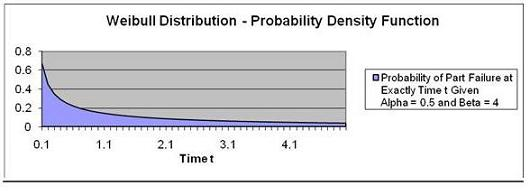The probability of part failure occurring at exactly Time t = 2 ("exactly at" indicates using the Probability Density Function) given that time to part failure is Weibull-distributed with a = 0.5  and ß = 4 is calculated is 8.7%.

Note that the graph point at Time t = 2.0 has a probability of 0.087.

Problem: Calculate the probability the a part will fail by time = 2 if the part's failure occurrence is Weibull-distributed and has a = 0.5 and ß = 4.

Time t = 2

α = Alpha = 0.5

ß = Beta = 4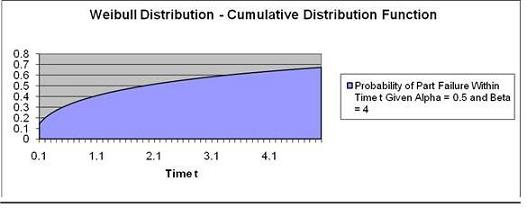We are determining the probability of part failure by time t = 2 so we are using the Cumulative Distribution Function.

The probability of part failure occurring at exactly Time t = 2 given that time to part failure is Weibull-distributed with α = 0.5 and ß = 4 is 50.6%.

This same Weibull Distribution problems as above are each solved in the Excel Statistical Master with only 1 Excel formula. You'll finally have a solid understanding of business statistics with the Excel Statistical Master.

# F - Distribution

The F Distribution is used to determine whether two groups have different variances. The F Distribution is normally used to develop confidence intervals and hypothesis tests. It is rarely used for modeling applications.

The F Distribution has 4 parameters: Χ21 (the calculated Chi-Square statistic for data group 1), Χ22 (the calculated Chi-Square statistic for data group 2), ѵ1 (the degrees of freedom of group 1), and ѵ2 (the degrees of freedom of group 2). An example of how the Chi-Square statistic is calculated from a group of data can be
found in the course module entitled "Chi-Square Independence Test."

The F Distribution is actually a family of distributions. Each different F Distribution has a unique combination of ѵ1 and ѵ2.

An individual F Distribution is actually the distribution of the F Statistic. The formula for the F Statistic is as follows:

F Statistic = ( Χ21 / ѵ1) / ( Χ22 / ѵ2)

As stated, the F Distribution is rarely used for modeling applications, but is often used for developing confidence intervals and hypothesis tests. Because of this, the most important use of a particular F Statistic is the calculation of its p Value. The p Value equals the percentage of total area under that unique F Distribution curve to the right of the given F statistic (and therefore the area in the outer curve tail
to the right of the F Statistic).

The p Value is compared with a, the required Level of Significance. If the p Value is less than α, then the two data groups are assumed to have different variances. If the p Value is greater than α, the two data groups are assumed to have equal variances.

ANOVA testing is used to judge whether three or more groups have the same mean (for example, same test scores) after each group has had a different treatment applied to it (for example, a different teaching method applied to each group).

If there no real differences between the groups being tested, one would expect that any measured differences between the groups would not be much different than measured differences between samples taken from within individual groups.

A F Ratio ( sometimes called an F Statistic) compares the differences between groups to the differences within groups.

Conceptually, the F Ratio can be thought of as how different the means of groups are relative to the variability within the groups. It might also be helpful to view the following explanation:

 F Ratio = Real Differences + Random Differences Between Groups Random Differences Within Groups

The actual definition of the F Ratio is as follows:
 F Ratio = Variance of the Group Means Mean of the Within-Group Variances

This is sometimes shortened to:
 F Ratio = Mean Square Between Groups Mean Square Within-Group

The larger the value of the F Ratio (sometimes called the F Statistic), the greater the likelihood that the difference between groups is due to Real Differences and not just due to chance (Random Differences).

The required degree of certainty (for example, we want to be at least 95% that the groups are different) determines how large the F Ratio has to be for us to be able to state that the groups are different.

The distribution of the F Ratio is called the F Distribution. The F Distribution is a family of distributions, each described by the following two parameters:

ѵ1 = Degrees of Freedom Between Groups

ѵ2 = Degrees of Freedom Within Groups

Critical F Values have been calculated for various degrees of certainty (99% certainty, 95% certainty, etc.) for each of the basic F Distributions. The general rule use to state whether real differences exists between groups for a given level of certainty is as follows:

General Rule:

If F Statistic (ѵ1 , ѵ2) > F Critical (ѵ1 , ѵ2)----> The different treatments affected the output

If F Statistic (ѵ1 , ѵ2) < F Critical (ѵ1 , ѵ2)
----> The different treatments did not affect the output

The F Statistic and F Critical are calculated using the same ѵ1 and ѵ2. If the F Statistic is greater than the F Critical that is calculated for a specific degree of certainty, we can state that groups are statistically different.

Calculating the F Statistic between two data sets involves a lot of work. A complete example of the calculation of the F Statistic between two data sets is shown at the end of the ANOVA module. Here, a hand-calculation of the F Statistic and p Value is performed to determine if there is a relationship between sales closing methods and sales results. The problem required a 95% Level of Certainty. The α (Level of Significance) was therefore equal to 0.05. Sales results and closing
methods are assumed to not be independent of each other because different closing methods are shown to produce different sales results.

A summary of that problem is as follows:

The problem requires determination of whether closing methods used have an affect on sales. Three sales groups were each required to use a different closing method for the entire test. The total sales results from each group were recorded. ANOVA analysis was employed to determine with a 95% Level of Certainty whether the choice of closing method affected the level of sales.

The ANOVA process breaks the data down in two ways for analysis. One grouping of data is labeled the "Between Groups" data. The other grouping of the
data is labeled the "Within Groups" data.

An F Statistic was calculated based upon these two groups of data. The calculated F Statistic was found to be greater than the F Critical Value that was based upon the 95% Level of Certainty. Therefore this implies that sales are not independent of closing method used. See the ANOVA module for the complete calculation.

A summary of the calculations is as follows:

"Between Groups" data grouping:
- Χ21 = Chi-Square StatisticGroup 1 = 72
- ѵ1 = degrees of freedomGroup 1 = 2

"Within Groups" data grouping:
- Χ22 = Chi-Square StatisticGroup 2 = 46
- ѵ2 = degrees of freedomGroup 2 = 9

F Statistic = ( Χ21 / ѵ1) / ( Χ22 / ѵ2)

= ( 72 / 2 ) / ( 46 / 9 )

= 7.04

 F Statistic(ѵ1 , ѵ2)  ---->  ѵ1 = DOF1 = 2, ѵ1 = DOF2 = 9 F Statistic (ѵ1=2,ѵ2=9) = (MS Between Groups) / (MS Within Groups) F Statistic (ѵ1=2,ѵ2=9) = 36 / 5.1 = 7.04

General Rule:

If F Statistic (ѵ1 , ѵ2) > F Critical (ѵ1 , ѵ2)----> The different treatments affected the output

If F Statistic (ѵ1 , ѵ2) < F Critical (ѵ1 , ѵ2)
----> The different treatments did not affect the output

The calculated F Statistic(ѵ1 = 2, ѵ2 = 9) = 7.04. This is greater than F Criticalα=0.05 (ѵ1 = 2, ѵ2 = 9) = 4.265. This indicates that there is less than a 5% chance that this result could have occurred if there was no difference in the effectiveness between the closing methods. Therefore, there is at least a 95% certainty that there is a real difference in effectiveness of the closing methods. The Null Hypothesis, which was therefore rejected, states that choice of closing methods does not affect sales.

The p Value (which can be quickly calculated with Excel) = 0.0144

0.0144 is less than a (0.05) so it is assumed that the two groups are not independent. Sales are therefore related to the closing method used because the variances are different.

If You Like This, Then Share It...